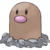# Catch rate (GO)

The calculation of catch rate is among the several mechanics that differ in Pokémon GO compared to the core series. Every species of Pokémon is assigned a base capture rate, a raw percentage that determines how difficult that species is to capture. This base rate is then modified by several factors, such as the wild Pokémon's Power Up level and how skillfully the player threw the Poké Ball, to determine the final catch rate.

For certain encounters, the encountered Pokémon is guaranteed capture on the first ball that hits, bypassing the catch rate calculation. These encounters include:

## CalculationThis section is incomplete.Please feel free to edit this section to add missing information and complete it. Reason: GO Battle League encounter multiplier?

Upon a thrown Poké Ball successfully hitting a wild Pokémon, the game will calculate $P$ , the probability of a successful capture.

$P=1-{\Bigl (}1-{\dfrac {baseCaptureRate}{2\times CPM}}{\Bigr )}^{modifier}$

where

• $baseCaptureRate$  is the base capture rate of the wild Pokémon's species.
• $CPM$  is the CP multiplier corresponding to the wild Pokémon's Power Up level.
• For standard wild encounters, a Pokémon's level ranges from 1 to 30 ($0.094\leq CPM\leq 0.7317$ ), or 5 to 35 if weather boosted ($0.29024988\leq CPM\leq 0.76156384$ ).
• For Raid Battles, a Pokémon's level is 20 ($CPM=0.5974$ ), or 25 if weather boosted ($CPM=0.667934$ ).
• For research task encounters, a Pokémon's level is always 15 ($CPM=0.51739395$ ).
• For Team GO Rocket encounters, a Pokémon's level is 8 ($CPM=0.37523559$ ), or 13 if weather boosted ($CPM=0.48168495$ ).
• For GO Battle League reward encounters, a Pokémon's level is always 20 ($CPM=0.5974$ ).

and

$modifier=Ball\times Berry\times Throw\times Curveball\times Medal\times Encounter$

where

• $Ball=$
• $Berry=$
• ${\textstyle Throw=2-r}$  if the Ball hits within the target ring, and 1 otherwise
• where $r={\dfrac {RingSize_{target}}{RingSize_{max}}}$ , hence $0
• for a Nice Throw, $1\leq Throw\leq 1.3$
• for a Great Throw, $1.3\leq Throw\leq 1.7$
• for an Excellent Throw, $1.7\leq Throw\leq 2$
• $Curveball=1.7$  if the Ball is spun before being thrown, and 1 otherwise
• $Encounter=$
• 2 if the Pokémon is encountered for completing Field, Special, or Timed Research tasks
• $x$  if the Pokémon is encountered during the bonus challenge of a Raid Battle
• $x$  is estimated to be between 1 and 2, increasing based on the number of Premier Balls that have already been thrown
• 1 if otherwise
• $Medal$  is based on the player's type-specific medals pertaining to the wild Pokémon's type, and it equals

When capturing Pokémon using a peripheral device, such as the Pokémon GO Plus or Poké Ball Plus, a regular Poké Ball will always be used with no bonus modifiers applied, except $Medal$ , if applicable.

## Target ring colors

The color of the target ring allows players to gauge the probability of a successful capture, factoring in all bonuses for $modifier$  except for $Throw$  and $Curveball$ . Switching Poké Balls or feeding Berries may change the color of the ring. The greener the ring, the higher the catch rate; the redder it is, the lower the catch rate. For Shadow Pokémon, the ring's color uses a base catch rate of 5%, while the true catch chance uses the species' base catch rate.

Catch rate by color
0%
10%
20%
30%
40%
50%
60%
70%
80%
90%
100%

## Critical captureThis section is incomplete.Please feel free to edit this section to add missing information and complete it. Reason: Chance of critical capture?

A critical capture is a rare phenomenon that was first observed in Pokémon GO in February 2017, alongside the release of Generation II Pokémon. Occasionally, a thrown Poké Ball will bypass catch rate calculations and guarantee a capture. Instead of shaking three times, a throw Poké Ball will only shake once, and the Pokémon will be confirmed caught. No additional bonuses are awarded for critical captures, but XP bonuses such as from Great or Curveball throws are still included.

## Examples

### Poké Balls and Berries

Consider a wild Pikachu with 343 CP, with an estimated Power Up level of 14. Pikachu has a base capture rate of 20% and a CP multiplier of 0.49985844 at level 14. Without any bonus modifiers, the catch rate will be ${\frac {20\%}{2\times 0.49985844}}\approx 20.006\%$ .

The following table shows what this Pikachu's catch rate would be if bonuses for Poké Balls and Berries were applied, assuming no bonuses from throws or medals.

### Throw technique

Consider a wild Tauros of 1252 CP, with an estimated Power Up level of 18. This means it will have a base capture rate of 30% and a CP multiplier of 0.56675452. Without any additional bonuses, the capture rate will be ${\frac {30\%}{2\times 0.56675452}}\approx 26.47\%$ .

The following table shows how the capture rate will be affected by throwing techniques, assuming no additional bonuses and only regular Poké Balls are used; throw bonus modifiers will be averaged between the highest and lowest possible values.

None Curveball
None
(×1.0)
26.47% 40.70%
Nice!
(×1.15)
29.78% 45.17%
Great!
(×1.5)
36.94% 54.34%
Excellent!
(×1.85)
43.38% 61.97%

### Medals

Let's now consider a wild Abra that has 670 CP, estimated to be Power Up level 20. Given that Abra has a base capture rate of 50% and its CP multiplier is 0.59740001, the catch rate without bonuses will be ${\frac {50\%}{2\times 0.59740001}}\approx 41.85\%$ .

The following table shows the effect on catch rate via medals for each Poké Ball type, assuming no other bonus modifiers.

## Trivia

• For all species, the base capture rate is approximately equal to the probability of capture for a Level 14 Pokémon with no $modifier$  bonuses.
• The highest theoretical $modifier$  that can be attained is ×47.6, which includes:
• Using an Ultra Ball (×2.0)
• Feeding a Golden Razz Berry (×2.5)
• Throwing a Curveball (×1.7)
• Making a perfect Excellent throw (×2.0)
• Having a platinum type medal bonus (×1.4)
• Encountering via Field, Special, or Timed Research (×2.0)